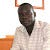# Introduction

1. What is the box model?
2. What are the main properties of a box?
3. What are the main types of boxes?
4. How to calculate the dimension of the box?
5. In what circumstances do margins collapse?
6. When content overflows.

# 1. What is the Box Model?